CBSE Sample Papers for Class 12 Chemistry Paper 3 are part of CBSE Sample Papers for Class 12 Chemistry. Here we have given CBSE Sample Papers for Class 12 Chemistry Paper 3.

## CBSE Sample Papers for Class 12 Chemistry Paper 3

 Board CBSE Class XII Subject Chemistry Sample Paper Set Paper 3 Category CBSE Sample Papers

Students who are going to appear for CBSE Class 12 Examinations are advised to practice the CBSE sample papers given here which is designed as per the latest Syllabus and marking scheme as prescribed by the CBSE is given here. Paper 3 of Solved CBSE Sample Paper for Class 12 Chemistry is given below with free PDF download solutions.

Time Allowed : 3 Hours

Max. Marks : 70

General Instructions

• All questions are compulsory.
• Question number 1 to 5 are very short answer questions and carry 1 mark each.
• Question number 6 to 10 are short answer questions and carry 2 marks each.
• Question number 11 to 22 are also short answer questions and carry 3 marks each.
• Question number 23 is a value based question and carry 4 marks.
• Question number 24 to 26 are long answer questions and carry 5 marks each.
• Use log table, if necessary. Use of calculators is not allowed.

Questions

Question 1.
Define zeta potential.

Question 2.
Name an oxoanion having oxidation number of metal equal to its group number.

Question 3.
Draw structure of 4-methoxy, N, N-dimethyl aniline.

Question 4.
Arrange according to increasing acidic strength- phenol, 2-methyl phenol, benzoic acid.

Question 5.
Name the type of semiconductor formed when Cu2O having metal deficiency defect.

Question 6.
[Fe(CN)6]4- and [Fe(H2O)6]2+ are of different colours in dilute solutions. Why?
OR
What is the co-ordination entity formed when excess of aqueous KCN is added to an aqueous solution of copper sulphate? Why is it that no precipitate of copper sulphide is obtained, when H2S(s) is passed through this solution?

Question 7.
(a) Draw the shape of BrF4.
(b) Arrange according to increasing boiling point HF, HCl, HI, HBr.

Question 8.
Define osmosis & osmotic pressure.

Question 9.
Show that in a first order reaction, time required for completion of 99.9% of reaction is 10 times of the half life (t1/2) of the reaction.

Question 10.
Write chemical reaction to affect the following change:
(a) Benzene to m-bromophenol
(b) Benzoic acid to aniline

Question 11.
X-ray diffraction studies show that copper crystallises in fee unit cell with cell edge of 3.6 x 10-8 cm and its density is 8.92 g/cm3. Calculate the atomic mass of copper.

Question 12.

1. What is the significance of leaching in the extraction of Al?
2. Describe a method for refining of nickel.
3. Which possesses more carbon-pig iron or cast iron?

Question 13.
Complete the following chemical equations:

1. XeF2 + H2O →
2. H2O + F2
3. Zn + HNO(dil.) →

Question 14.

1. Find co-ordination number of metal ion in [Co(ox)2Cl2]+?
2. Which will react with ethylene diamine-ris [Pt(NH3)2Cl2] or trans [Pt(NH3)2Cl2]?
3. What is the role of co-ordination compounds in analytical chemistry. Give one example.

Question 15.
(a) Chlorobutane has higher boiling point than f-butyl chloride. Explain
(b) Arrange according to increasing SN2 reactivity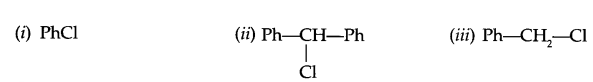(c) Give reason, why do alcoholic KOH gives alkene on reaction with haloalkane?

Question 16.

1. Why do all digestive tablets contain salt?
2. Arrange according to increasing coagulating power-Na3PO4, NaCl, sugar.
3. Define electrophoresis.

Question 17.
Calculate the mass of a non volatile solute AB2 (molar mass 60 g/mol) which should be dissolved in 5 litre of water to boil it at 108°C, if Kb for water is 0.52 K Kg mol-1. (The solution is 80% dissociated in H2O).
OR
Calculate the osmotic pressure in pascals exerted by a solution prepared by dissolving 1.0 g of polymer of molar mass 1,85,000 g per mole in 450 ml of water at 37°C.

Question 18.
Distinguish between the following pair with a suitable chemical test:

1. Phenol and benzyl alcohol
2. Propanmine and propanal
3. Methanol and propanal

Question 19.
Illustrate the following reaction with a suitable chemical reaction:

1. Carbylamine reaction
2. Crossaldol condensation
3. Sandmeyer reaction

Question 20.

1. Name three type of RNA.
3. Name the deficiency disease caused by vitamin ‘D’.

Question 21.
(a) If slope of this line is -1.25 × 104 K, what will be value of Ea?
(b) If intercept of the line on Y-axis is 14.34 find arrhenius constant ‘A’.

Question 22.
Write monomer units with structure and name for the following polymers:
(a) PTEE
(b) Buna-N
(c) Dacron

Question 23.
In a holiday, Neelam wanted to prepare some halwa for her husband who is diabetic. She does not want to add sugar to halwa, so she wants to use aspartame as artificial sweetner. Her daughter Tanya, a student of class XII chemistry suggested not to use aspartame.

1. Why do Tanya suggested her not to use aspartame?
2. Suggest a suitable artificial sweetner for making Halwa.
3. In which type of food aspartame can be used.
4. Mention the values associated with the suggestion of Tanya.

Question 24.
(i) State the relationship amongst cell constant of a cell, resistance of the solution in the cell and conductivity of the solution. How is molar conductivity of a solution related to conductivity of its solution?
(ii) A voltaic cell is set up at 25°C with the following half cell Al/Al3+ (0.001 M) and Ni/Ni2+ (0.50M). Calculate the cell voltageOR
(i) Calculate the potential of hydrogen electrode in contact with a solution whose pH is 10.
(ii) State Faraday’s laws of electrolysis. How much charge in terms of Faraday, F is required for reduction of 1 mol Cr2O2-7 to Cr3+.

Question 25.
(i) Complete the following chemical reactions:
(a) kMnO4 $$\underrightarrow { Heat }$$
(b) Cr2O2-7 + H2S + H+
(ii) Describe the preparation of potassium dichromate from chromate ore with chemical equations involved. What is the effect of increasing pH on a solution of potassium dichromate?
OR
Explain the following:

1. Copper (I) ion is not stable in an aqueous solution.
2. Transition metals in general acts as good catalysts.
3. Lanthanoid contraction is less than actinoid contraction.
4. Melting point of Fe is more than Mn.
5. Name the lanthanoid having +4 oxidation state and which acts as oxidising agent.

Question 26.
Two moles of an organic compound ‘A’ (C7H6O) on treatment with a strong base gives two compound ‘B’ and ‘C’. ‘B’ on oxidation with alkaline KMnO4 gives ‘C’. B on reaction with ‘C’ in the presence of cone. H2SO4 gives fruity smelling compound ‘D’. Write all the reactions and draw structures of A, B, C & D.
ORZeta potential: Adsorption of ions on particles of dispersed phase leads to the formation of electrical double layer. The potential difference between the fixed layer and the diffused layer of opposite charges is called zeta potential or electrokinetic potential.

MnO4, Cr2O2-7, CrO2-4 (any one).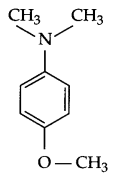2-methyl phenol < phenol < benoic acid (Increasing acidic strength)

p-type semiconductor

Explanation according to VBT : Iron is in + 2 state and have 3d6 configuration in both the complexes, but [Fe(CN)6]4- is an inner orbital-complex so no unpaired electron is there and as a result it is colourless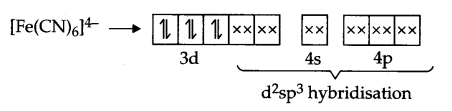On the other hand [Fe(H2O)6]2+ is an outer orbital-complex, hence there is unpaired electrons and the complex is coloured.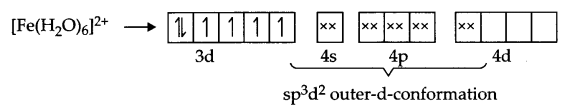According to crystal field theory, CN is a strong field ligand but H2O is a weak field ligand, hence t2g eg configuration is as follows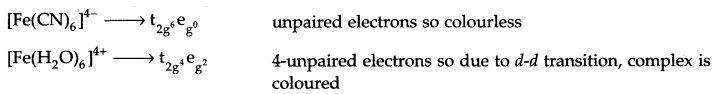OR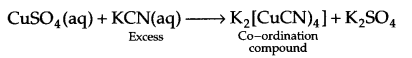since Cu is within the co-ordination sphere, so it will not get precipitated as CuS on passing H2S(s).(b) HCl < HBr < HI < HF (Increasing boiling point)

• Osmosis : Movement of solvent particles from low concentration solution to higher concentration solution through a semipermeable membrane is known as osmosis.
• Osmotic pressure : The pressure applied on higher concentration side of a semipermeable membrane separating solutions of different concentration, so that there is no net flow of solvent on either side of membrane is known as osmotic pressure.

For a first order reactionfor 99.9% completion of a reaction, if [A]0 = 100, then
[A]t = 100 – 99.9 = 0.1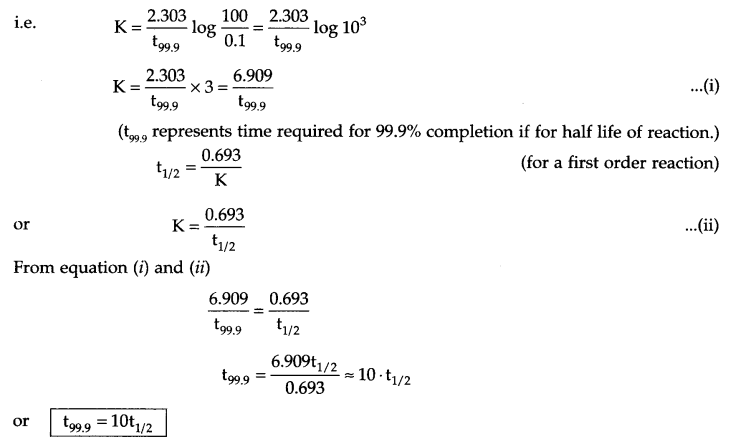(a) Benzene to m-bromophenol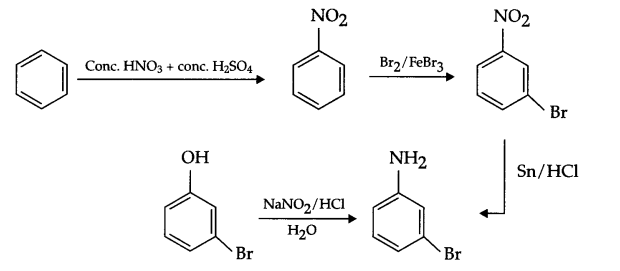(b) Benzoic acid to anilineFrom question Z = 4, a = 3.6 x 10-8 cm, d = 8.92 g/cm3, λ = ?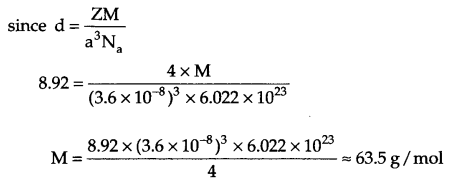1. Leaching with NaOH(aq) makes soluble complex Al2O3 and leaving behind all the impurities.
Al2O3 . xH2O + NaOH (aq) → Na [Al(OH)4]
[from this soluble complex on passing CO2, Al(OH)3 gets precipitated from which pure Al2O3 can be obtained]
2. Mond’s process is used for refining of nickel in this method Ni converted to volatile complex which breaks down on further heating.3. Pig iron (4% C) possesses more carbon content than cast iron (3% C)

1. 2XeF(s) + 2H2O(l) → 2Xe(g) + 4HF(aq) + O2(g)
2. 2H2O (l) + 2F2(g) → 4HF (aq) + O2(g)
3. Zn + HNO3 (dil) → 4Zn(NO3)2 + 5H2O + N2O

1. Co-ordination number = 6 (∵ ox(Oxilato-bidentate ligand)
2. Ethylene diamine, a bidentate ligand can react only with cis isomer, so cis is [Pt(NH3)2 Cl2] is the correct answer.
3. Role of co-ordination compound in analytical chemistry
• EDTA for estimation of hardness of water
• DMG for analysis/confirmation of Ni2+ ion
• Confirmation of chloride ion by dissolving AgCl in NH3 solution.

(a) Chlorobutane has larger surface area than compact butyl chloride and as a result, more intermolecular forces in chlorobutane and it has higher boiling point.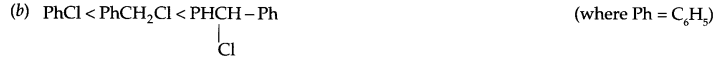(c) KOH + C2H5OH → C2H2OK+ + H2O
Ethoxide ion forms in presence of alcohol is a stronger base than OH (hydroxyl) ion, hence it can remove β-hydrogen and leads to the formation of alkene.

1. Salt is a strong electrolyte, hence coagulate food particles easily and so helps in easy digestion.
2. Sugar < NaCl < Na3PO4
3. The movement of colloidal particles under an applied electric potential is called electrophoresis.

From question
ΔTb = 108° – 100° = 8°C
= 381 – 373 = 8 K
mass of solvent = 5 litre = 5000 g
Kb = 0.52 K kg mol-1, molar mass of solute = 60 g/mol
AB2 is 80% dissociated, so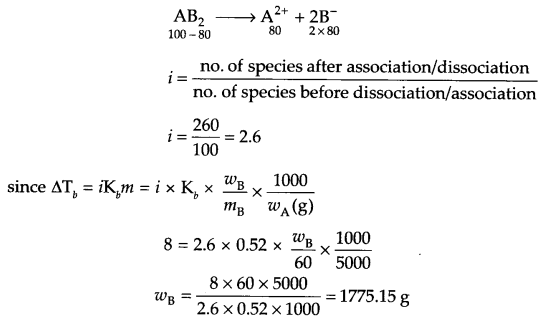OR
It is given that
wB= 1 g, mB = 1,85,000 g/mol, wA = 450 mL
T = 37 + 273 = 310K, π = ?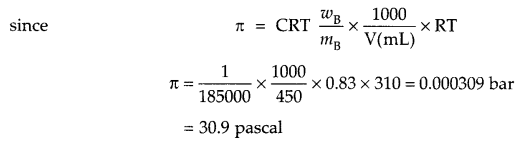(a)

 Reagent Phenol Benzyl alcohol Neutral FeCl3 Dark grey/violet colour appears No reaction

(b)

 Reagent Propanmine Propanal I2 + NaOH heat Yellow precipitate will form No yellow Precipitation

(c)

 Reagent Methanol Propanal KMnO4(alk) HCHO + KMnO4 → CO2 + H2O + Mn2+ CO2 gas is evolved Acid will form, evolve CO2 on adding NaHCO3

1. Carbylamine reaction :2. Cross aldol condensation: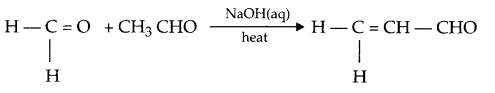3. Sandmeyer reaction: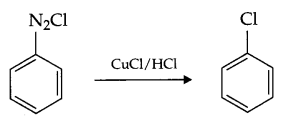1. Three type of RNA
• m-RNA
• t-RNA
• r-RNAThe linkage (amide linkage) present between two amino acids is known as peptide linkage in protein chemistry.
3. Deficiency disease caused by vitamin-D → Rickets and osteomalacia.

(a) From Arrhenius relation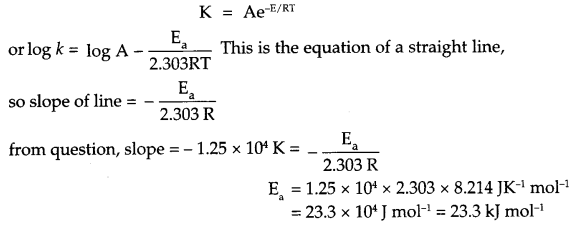(b) Since intercept on y-axis is log A, so
log A = 14.34
A = antilog 14.34
A = 2.188 x 1014 s-1.

(a) PTEE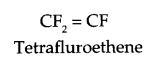(b) Buna-N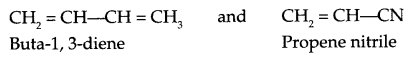(c) Dacron :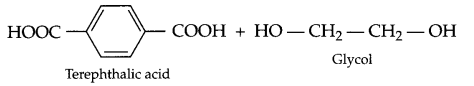1. Since aspartame is unstable at cooking temperature, so Tanya suggested her mother not to use it.
2. Sucralose or Alitame
3. Aspartame can be used for cold food items.
4. Knowledge of chemistry, concern for family.

(i) Relationship between cell constant, resistance and conductivity of a cell:(ii) Since Al3+/Al reduction potential has more -ve value as it will act as anode and nickel will act as cathode. The cell will be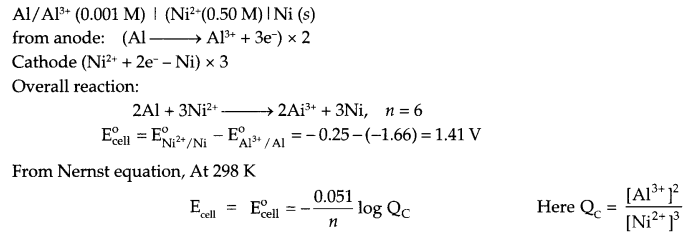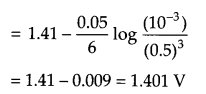OR
Since pH of solution is 10, so [H+] = 10
From Nemst equation at 298 K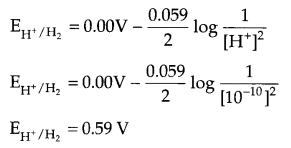(ii) Faraday’s first law : The amount of chemical reaction which occurs at any electrode during electrolysis by a current is proportional to the quantity of electricity passed through the electrolytic solution or melt.
Faraday’s second law : The amount of different substances liberated by the same quantity of electricity passing through the electrolytic solution are proportional to their chemical equivalent weights.
Number of Faraday’s required for Cr2O2-7 to Cr3+ is 6 F.

(i) (a) 2KMnO4 $$\underrightarrow { Heat }$$ K2MnO4 + MnO2 + O2
(b) Cr2O2-7 + 3H2S + 8H+ → 2Cr3+ + 3S + 7H2O
(ii) Preparation of potassium dichromate:Effect of increasing pH :
Dichromate ion will convert to form chromate ion as follows :OR

1. Cu+ in aqueous solution undergoes disproportionation, i.e.
2Cu+(aq) → Cu2+(aq) + Cu(s)
The E° value for this reaction is favourable (due to high hydration energy for Cu2+ ion)
2. Catalytic property of transition metals is due to their ability of adopting multiple oxidation state and to form complexes.
3. Shielding effect of 4f electrons is more as compared to 5f orbital electrons and hence lanthanoid contraction is less than actinoid contraction.
4. The size of iron is smaller than manganese which compensate for one less impaired electron present in iron in comparison to manganese, hence M-M bond in iron is stronger than Mn and as a result iron has higher melting point than Mn.
5. Lanthanoids with +4 oxidation state are → Ce4+, Gd4+, HO4+, Dy4+.
These acts as oxidising agent to acquire +3 oxidation state like other elements of their group.